NEURONS SPIKE BACK ：The Invention of Inductive Machines and the Artificial Intelligence Controversy

Antoine MAZIÈRES，Dominique CARDON 。0+3+0+

### 相关内容1+9+0+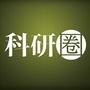0+3+0+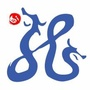0+9+0+0+9+0+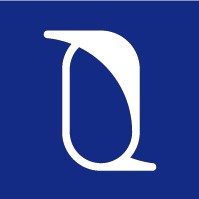0+3+0+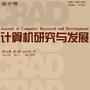0+5+0+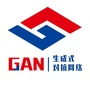0+4+0+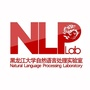0+3+0+0+6+0+0+3+0+
Top